# Commercial Math

Go back to  'Maths'

 1 Introduction to Commercial Math 2 Money 3 Ratio 4 Proportion 5 Percentages 6 Unitary Method 7 Profit and Loss 8 Simple Interest 9 Compound Interest 10 Discounts 11 Taxes

## Introduction

Commercial math focuses on those aspects of mathematics that are used in everyday life.

Consider an example;

Avantika, your friend goes to a stationary shop to buy a notebook.

The shopkeeper tells her that 12 notebooks will cost Rs 200.

She has to calculate the cost of 1 notebook. This requires an understanding of the unitary method.

Let’s take another example;

Rithvik goes to buy a cricket bat with his mother.

One shop offers him a 25% discount on the bat. The other shop offers a free cricket ball with the bat.

Rithvik wants to help his mother select the best deal. He should know how to calculate percentages and also understand the concept of discounts.

In both these examples, we make use of commercial math concepts.

You can go ahead and explore all important topics in Commercial Math by selecting the topics from this list below:

 Money Profit and Loss Ratios Simple Interest Proportion Compound Interest Percentages Discounts Unitary Method Taxes

## Money

Money is what makes the world go round, and mathematics is the centripetal force that governs it. From simple transactions to banking, taxes and discounts, everything that involves money, involves math!

Example: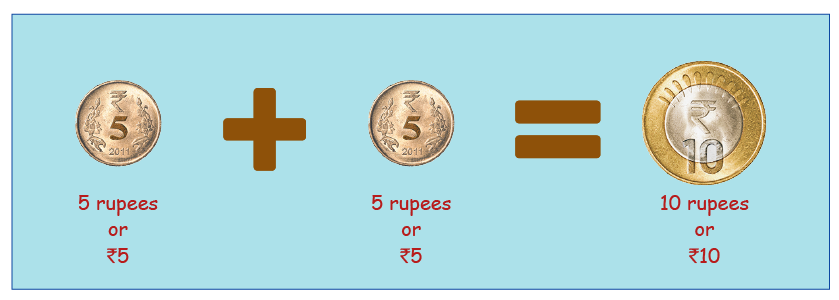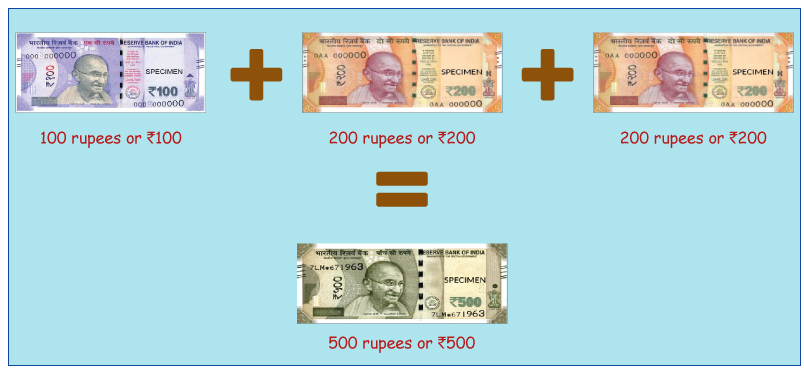Money is a medium of exchange. We pay coins and notes in order to get services and commodities. You can now learn more about the process of Identifying Denominations and the concept of Decomposing Money.

## Ratio

A ratio tells us how much of the proportion of one thing to the other. The sequence (or order) in which the numbers in a ratio are written matters.

Example:## Proportion

When two ratios are equivalent, they are said to be in proportion.

Example: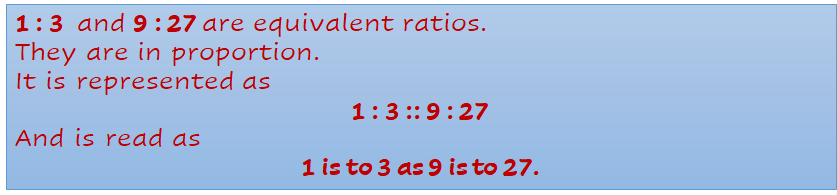Check out the examples of Proportion to understand the concept better.

## Percentages

Percent means “out of $$100$$”. If $$20$$ out of $$100$$ boxes are shaded, then we say that $$20$$ percent or $$20%$$ of the boxes are shaded.

Example:You can now learn how to calculate Percentages and its applications with the help of examples.

## Unitary Method

The unitary method, in essence, is all about finding the “per unit value”. The unitary method is a technique for solving a problem by first finding the value of a single unit, and then finding the necessary value by multiplying the single unit value.

Example: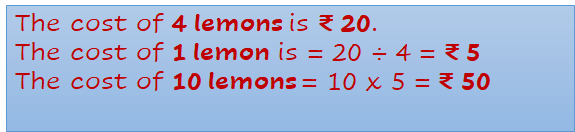You can try solving some of the examples of Unitary Method to understand the methods used in solving such problems.

## Profit and Loss

Profit is the gain that is incurred when the Selling Price (SP) of a commodity is greater than its Cost Price (CP).

Loss is incurred when the Selling Price (SP) of a commodity is less than its Cost Price (CP).

Example: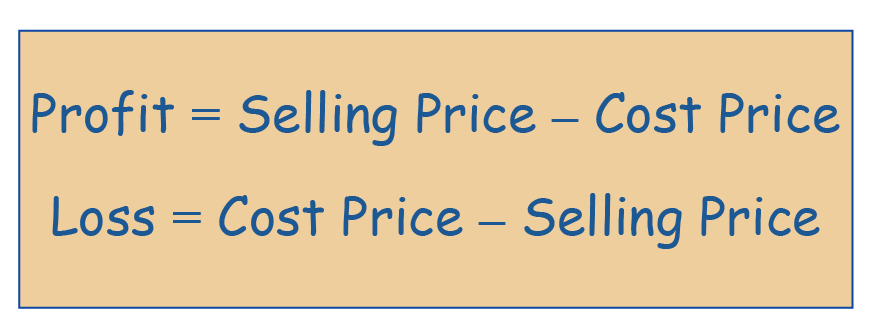Profit and Loss are the basic driving forces of the market, and here you will understand how to wield the power of mathematics and apply it to the commercial world.

## Simple Interest

Simple Interest is the interest that is calculated on the Principal Amount on a monthly, quarterly, or annual basis.

Example: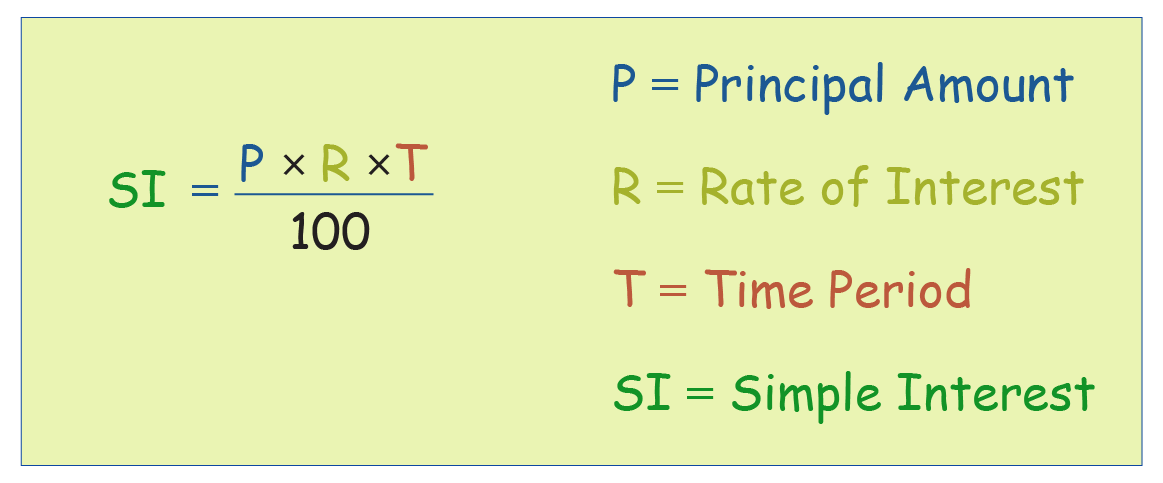Ever borrowed money from a friend or relative and they said that you would have to return the amount after a year with 10% interest? The concept of Simple Interest will help you easily calculate the total amount you would need to repay them.

## Compound Interest

Compound Interest is the interest that is calculated on the Principal Amount and the Interest that is obtained from the previous term.

Example: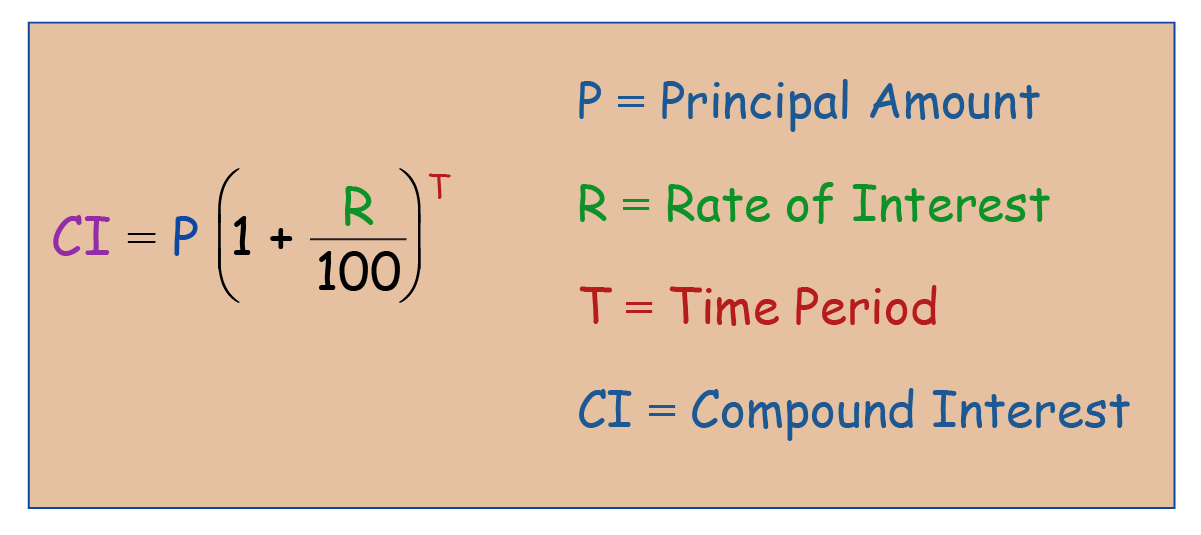Compound Interest is usually charged by banks and insurance companies on the amount of loan taken by us from them.

## Discounts

Discount is a reduction in the price of a commodity. It is the difference between the selling price and marked price.

Example: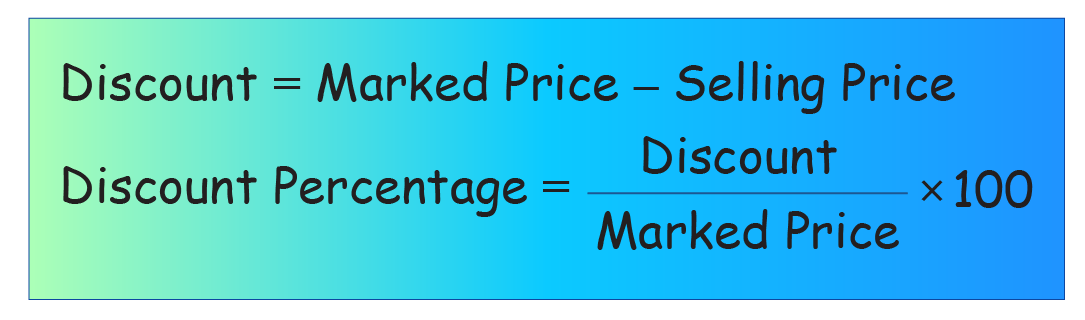Discounts are probably the most frequent terms that come up during a shopping trip or any purchase that we make since they directly affect the amount of money involved.

## Taxes

The government charges a fee on every item that is sold. This fee is meant to be spent on public welfare and is known as tax. The tax charged is always a percentage of the SP.

Example: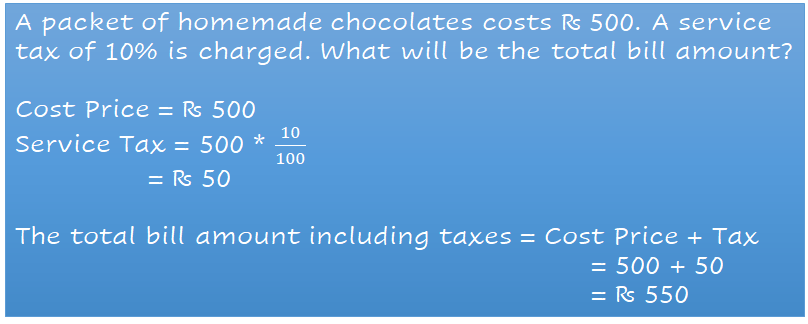You must have heard words like income tax, GST, service tax etc in daily conversations. Let’s now try and understand how Taxes are levied on products

Learn from the best math teachers and top your exams

• Live one on one classroom and doubt clearing
• Practice worksheets in and after class for conceptual clarity
• Personalized curriculum to keep up with school

0